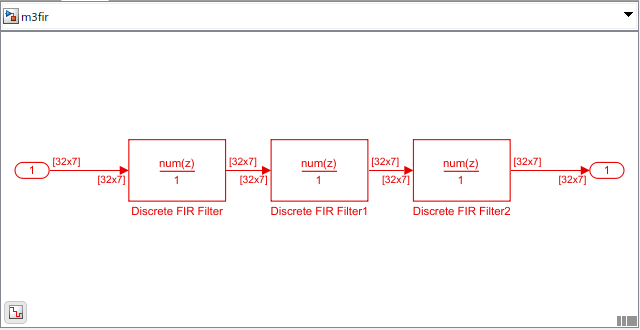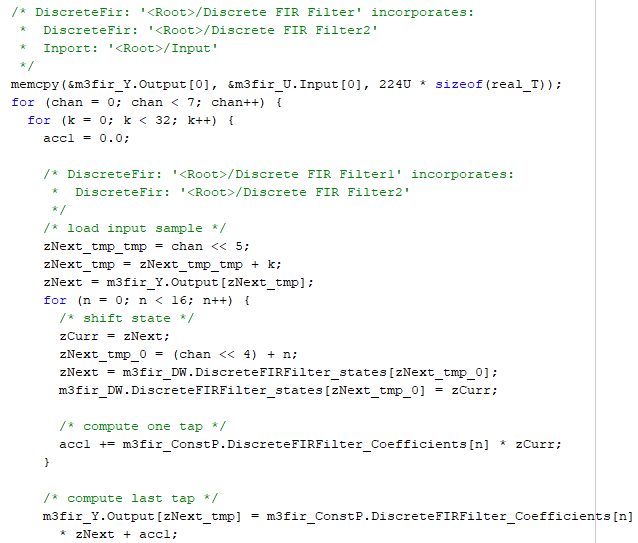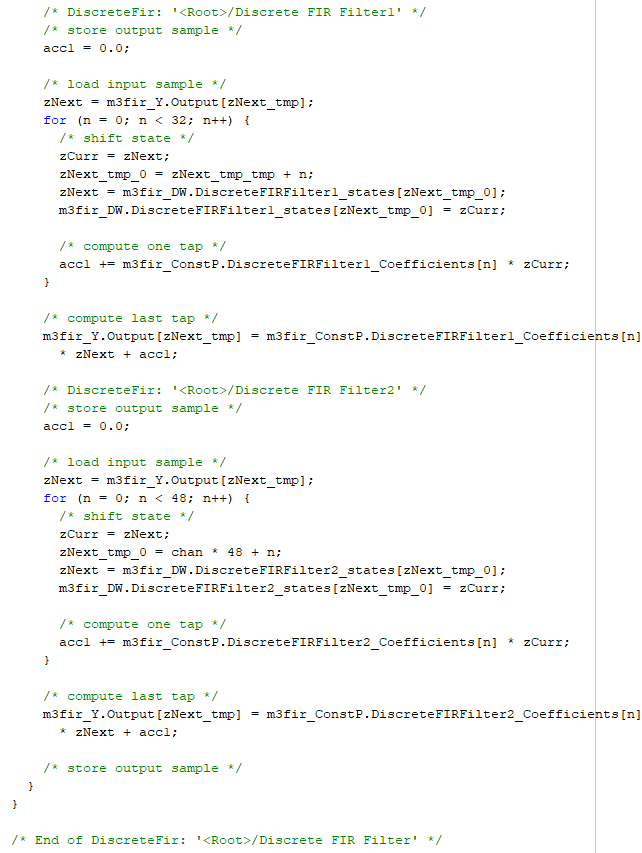## In-Place Memory Optimization

In-place optimization is a memory optimization technique that uses a single buffer, that is, the same memory to store the input and output data. Every time there is an intermediary output value in the algorithm, the same buffer is overwritten to store this value. This technique optimizes the memory usage and generates code that uses very less memory.

These following features in DSP System Toolbox™ support in-place memory optimization.

To illustrate the technique of in-place optimization, consider this model that contains a sequence of three, connected Discrete FIR Filter blocks. Each block filters the input it receives and generates an output that is of the same size as the input.When you generate code from such a model, you can see the in-place optimization in the generated code.

This section shows the in-place optimized generated code. The three `for` loops with the iteration index n correspond to the respective Discrete FIR Filter block in the Simulink® model. In each of these three `for` loops, the filter output is computed and stored in the m3fir_Y.Output[] buffer. The m3fir_Y.Output[] buffer rewrites its value every time the Discrete FIR Filter block has an updated output.The generated code reuses the output buffer and hence is efficient and uses less memory.

When you generate code from a MATLAB® algorithm containing a sequence of `dsp.FIRFilter` objects, you see a similar optimization in the generated code.

## SupportGet trial now